2020
04-29

# php中RabbitMQ的使用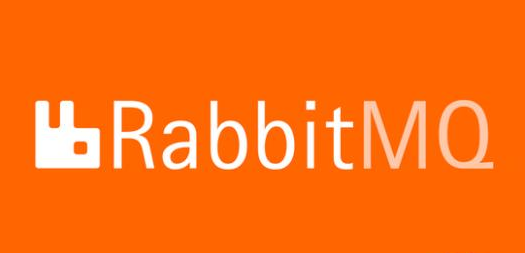####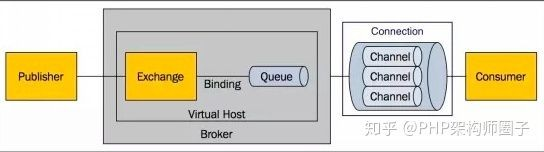rmq简单来说就是一个（生产/消费）的模型结构。消息生产者把数据丢到队列中，消息消费者从队列中取出数据进行逻辑处理。

rmq（消息队列）主要提供了三个概念（中间件？）来确保消息的分发，Exchange（交换机）、RoutingKey（路由）、Queue（队列）

Exchange 所有生产消息的入口都是到交换机这里。

exchange通过进来的路由（RoutingKey），去和已binding的规则进行匹配，找到指定的队列。

RoutingKey 我的理解，这里相当于一把钥匙。而binding的操作相当于一把锁头。

Queue 消息的存放区域，等到消费者来取。

Binding Exchange和Queue之间的一个绑定。

exchange类型

RabbitMQ提供了四种Exchange类型

1. direct
2. fanout
3. topic

Direct Exchange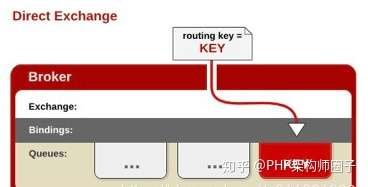direct 的规则比较简单。

Fanout Exchange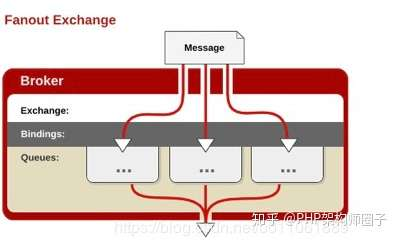fanout 类型则更简单一些。 只要exchange和队列做了绑定。发布的消息都会到队列中去。

Topic Exchange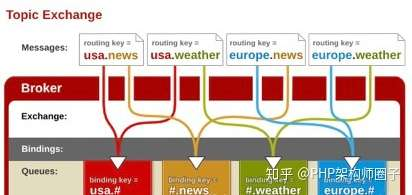```\$mqModel = new Rabbitmq();   // 初始化（rmq连接操作）
\$newResult = ['tom','bill','jack'];
if (\$mqModel) {
\$mqRoute = 'push_data_to_crm_routing';  // 路由
\$mqExchange = 'push_data_to_crm_exchange';  // 交换机
\$mqQuery = 'push_data_to_crm_queue';  // 队列
// 建立连接，设置交换机，设置队列
\$mqModel->setChannel()->setExchange(\$mqExchange,AMQP_EX_TYPE_DIRECT,AMQP_DURABLE)->setQueue(\$mqQuery,AMQP_DURABLE,\$mqExchange,\$mqRoute);
foreach (\$newResult as \$k => \$v){
\$push_data = \$v;
\$mqModel->publishMessage(\$push_data,\$mqRoute); // 消息推送
}
}```

```\$mqModel = new Rabbitmq();
// \$mqRoute = 'push_data_to_crm_routing'; 消费者用不上路由，因为不需要指定。 只要想取队列，消费即可。
\$mqExchange = 'push_data_to_crm_exchange';
\$mqQuery = 'push_data_to_crm_queue';
\$mqModel->setChannel()->setExchange(\$mqExchange,'', AMQP_PASSIVE)->setQueue(\$mqQuery, AMQP_PASSIVE);
\$zmq->consume(function(\$msg){
var_dump(\$msg);
return true;
});```

```//设置交换机
public function setExchange(\$changeName = '', \$changeType = '', \$flags = false) {
\$errorMsg = '';
try{
if(!\$this->channel){
throw new \AMQPQueueException("Error channel on method setExchange", 1);
}
\$this->exchange = new \AMQPExchange(\$this->channel);
if(\$changeName){
\$this->changeName = \$changeName; // 交换机名称
\$this->exchange->setName(\$changeName); // 设置名称
\$changeType = \$changeType ? \$changeType : AMQP_EX_TYPE_DIRECT;  // 交换机类型
}else{
\$this->changeName = '';
}
if(\$changeType){
\$this->changeType = \$changeType;
\$this->exchange->settype(\$changeType);  // 设置交换机类型
}else{
\$this->changeType = '';
}
if(\$flags){
\$this->exchange->setFlags(\$flags);  //交换机标志
}
if(\$changeType || \$flags){
\$this->exchange->declareExchange();  // 创建
}
} catch(AMQPQueueException \$ex) {
\$errorMsg = "AMQPQueueException error exchange: {\$ex->getMessage()},\r\nline: {\$ex->getLine()}\r\n";
} catch(Exception \$ex) {
\$errorMsg = "Exception error exchange:  {\$ex->getMessage()},\r\nline: {\$ex->getLine()}\r\n";
}
if(\$errorMsg){
throw new Exception(\$errorMsg, 1);
}
return \$this;
}

// 设置队列
public function setQueue(\$queueName = '', \$flags = '', \$exchange_name = '', \$routing_key = '', \$arguments=[] ){
\$errorMsg = '';
try{
if(!\$this->channel){
throw new \AMQPQueueException("Error channel on method setQueue", 1);
}
\$this->queue = new \AMQPQueue(\$this->channel);
if(!\$queueName){
return false;
}
\$this->queueName = \$queueName;  // 队列名称
\$this->queue->setName(\$queueName);
if(\$flags){
\$this->queue->setFlags(\$flags);  // 队列标志。与消息持久化有关。 这篇文字不涉及这一块的说明
}
if(is_array(\$arguments) && !empty(\$arguments)){
\$this->queue->setArguments(\$arguments);  // 参数配置
}
\$this->queue->declareQueue();  // 创建一个队列
\$exchange_name = \$exchange_name === false ?
'' :
(\$exchange_name === true || !\$exchange_name ? \$this->changeName : \$exchange_name);

\$routing_key = \$routing_key ? \$routing_key : \$this->queueName;

if(\$exchange_name && \$routing_key ){
\$this->queue->bind(\$exchange_name, \$routing_key);  // 交换机和队列的绑定操作
}

} catch(AMQPQueueException \$ex) {
\$errorMsg = "AMQPQueueException error queue: {\$ex->getMessage()},\r\nline: {\$ex->getLine()}\r\n";
} catch(Exception \$ex) {
\$errorMsg = "Exception error queue:  {\$ex->getMessage()},\r\nline: {\$ex->getLine()}\r\n";
}

if(\$errorMsg){
throw new Exception(\$errorMsg, 1);
}

return \$this;
}

// 发布消息
public function publishMessage(\$message = '', \$routing_key = '', \$flags = AMQP_NOPARAM, \$attributes = []){
if(!\$message){
return false;
}
\$routing_key = \$routing_key ? \$routing_key : \$this->queueName;
// 发布消息，带有路由key。如果需要，则会用于关联。
\$this->exchange->publish(\$message, \$routing_key, \$flags, \$attributes);
return true;
}

// 消费
public function consume(\$callback = null, \$qos = 0, \$isAct = true){
if(\$qos){
\$this->channel->qos(0, \$qos);
}
\$errorMsg = '';
try{
if(!\$this->queue){
throw new \AMQPQueueException("Error queue on method consume", 1);
}
\$this->callBackFnc = \$callback;
\$this->isAct = \$isAct;
\$callback = function(\$envelope, \$queue){
if(is_callable(\$this->callBackFnc)){
call_user_func(\$this->callBackFnc, \$envelope->getBody());
if(\$this->isAct){
\$queue->ack(\$envelope->getDeliveryTag());
}else{
\$queue->nack(\$envelope->getDeliveryTag());
}
}
};
\$this->queue->consume(\$callback);
} catch(AMQPQueueException \$ex) {
\$errorMsg = "AMQPQueueException error queue: {\$ex->getMessage()},\r\nline: {\$ex->getLine()}\r\n";
} catch(Exception \$ex) {
\$errorMsg = "Exception error queue:  {\$ex->getMessage()},\r\nline: {\$ex->getLine()}\r\n";
}
if(\$errorMsg){
throw new \Exception(\$errorMsg, 1);
}
}```

#### 执行结果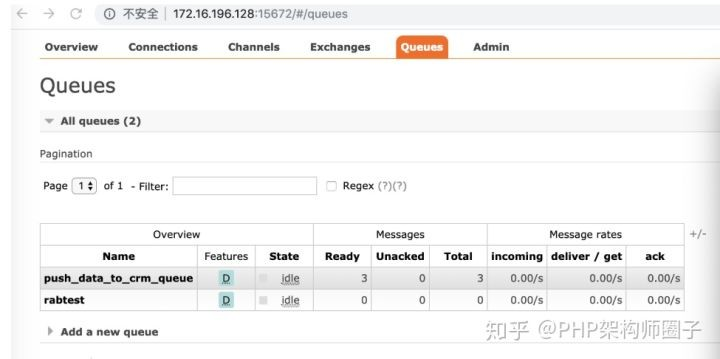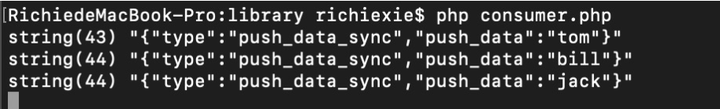#### 结尾